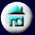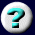11. Conservation of Mass,       Charge, and Energy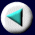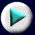Mass and Energy: Nuclear Reactions
 With nuclear reactions, the energies involved are so great that the changes in mass become easily measurable. One no longer can assume that mass and energy are conserved separately, but must take into account their interconversion via Einstein's relationship, E = mc. If mass is in grams and the velocity of light is expressed as c = 3 x 10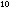cm sec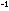, then the energy is in units of g cmsec, or ergs. A useful conversion is from mass in amu to energy in million electron volts (MeV): 1 amu = 931.4 MeV What holds a nucleus together? If we attempt to bring two protons and two neutrons together to form a helium nucleus, we might reasonably expect the positively charged protons to repel one another violently. Then what keeps them together in the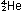nucleus? The answer, as we mentioned in Chapter 2, is that a helium atom is lighter than the sum of two protons, two neutrons, and two electrons. Some of the mass of the separated particles is converted into energy and dissipated when the nucleus is formed. Before the helium nucleus can be torn apart into its component particles, this dissipated energy must be restored and turned back into mass. Unless this energy is provided, the nucleus cannot be taken apart. This energy is termed the binding energy of the helium nucleus.
Page 21 of 37